# Introduction

Welcome to the StratifiedMedicine R package. The overall goal of this package is to develop analytic and visualization tools to aid in stratified and personalized medicine. Stratified medicine aims to find subsets or subgroups of patients with similar treatment effects, for example responders vs non-responders, while personalized medicine aims to understand treatment effects at the individual level (does a specific individual respond to treatment A?). Development of this package is ongoing.

Currently, the main algorithm in this package is “PRISM” (Patient Response Identifiers for Stratified Medicine; Jemielita and Mehrotra 2019 in progress). Given a data-structure of $$(Y, A, X)$$ (outcome, treatments, covariates), PRISM is a five step procedure:

1. Estimand: Determine the question or estimand of interest. For example, $$\theta_0 = E(Y|A=1)-E(Y|A=0)$$, where A is a binary treatment variable. While this isn’t an explicit step in the PRISM function, the question of interest guides how to set up PRISM.

2. Filter (filter): Reduce covariate space by removing variables unrelated to outcome/treatment. Formally: $filter(Y, A, X) \longrightarrow (Y, A, X^{\star})$ where $$X^{\star}$$ has potentially lower dimension than $$X$$.

3. Patient-level estimate (ple): Estimate counterfactual patient-level quantities, for example the individual treatment effect, $$\theta(x) = E(Y|X=x,A=1)-E(Y|X=x,A=0)$$. Formally: $ple(Y, A, X^{\star}) \longrightarrow \hat{\mathbf{\theta}}(X^{\star})$ where $$\hat{\mathbf{\theta}}(X^{\star})$$ is the vector of patient-level estimates.

4. Subgroup model (submod): Partition the data into subsets of patients (likely with similar treatment effects). Formally: $submod(Y, A, X^{\star}, \hat{\mathbf{\theta}}(X^{\star})) \longrightarrow \mathbf{S}(X^{\star})$ where $$\mathbf{S}(X^{\star})$$ is a distinct set of rules that define the $$k=0,...,K$$ discovered subgroups, for example $$\mathbf{S}(X^{\star}) = \{X_1=0, X_2=0\}$$. Note that subgroups could be formed using the observed outcomes, PLEs, or both. By default, $$k=0$$ corresponds to the overall population.

5. Parameter estimation and inference (param): For the overall population and discovered subgroups, output point estimates and variability metrics. Formally: $param(Y, A, X^{\star}, \hat{\mathbf{\theta}}(X^{\star}), \mathbf{S}(X^{\star}) ) \longrightarrow \{ \hat{\theta}_{k}, SE(\hat{\theta}_k), CI_{\alpha}(\hat{\theta}_{k}), P(\hat{\theta}_{k} > c) \} \text{ for } k=0,...K$ where $$\hat{\theta}_{k}$$ is the point-estimate, $$SE(\hat{\theta}_k)$$ is the standard error, $$CI_{\alpha}(\hat{\theta}_{k})$$ is a two (or one) sided confidence interval with nominal coverage $$1-\alpha$$, and $$P(\hat{\theta}_{k} > c)$$ is a probability statement for some constant $$c$$ (ex: $$c=0$$). These outputs are crucial for Go-No-Go decision making.

Ultimately, PRISM provides information at the patient-level, the subgroup-level (if any), and the overall population. While there are defaults in place, the user can also input their own functions/model wrappers into the PRISM algorithm. We will demonstrate this later.

# Example: Continuous Outcome with Binary Treatment

Consider a continuous outcome (ex: % change in tumor size) with a binary treatment (study drug vs standard of care). The estimand of interest is the average treatment effect, $$\theta_0 = E(Y|A=1)-E(Y|A=0)$$. First, we simulate continuous data where roughly 30% of the patients receive no treatment-benefit for using $$A=1$$ vs $$A=0$$. Responders vs non-responders are defined by the continuous predictive covariates $$X_1$$ and $$X_2$$ for a total of four subgroups. Subgroup treatment effects are: $$\theta_{1} = 0$$ ($$X_1 \leq 0, X_2 \leq 0$$), $$\theta_{2} = 0.25 (X_1 > 0, X_2 \leq 0)$$, $$\theta_{3} = 0.45 (X_1 \leq 0, X2 > 0$$), $$\theta_{4} = 0.65 (X_1>0, X_2>0)$$.

library(ggplot2)
#> Warning: package 'ggplot2' was built under R version 3.5.2
library(dplyr)
#> Warning: package 'dplyr' was built under R version 3.5.2
#>
#> Attaching package: 'dplyr'
#> The following objects are masked from 'package:stats':
#>
#>     filter, lag
#> The following objects are masked from 'package:base':
#>
#>     intersect, setdiff, setequal, union
library(partykit)
#> Warning: package 'partykit' was built under R version 3.5.2
#> Warning: package 'libcoin' was built under R version 3.5.2
#> Warning: package 'mvtnorm' was built under R version 3.5.2
library(StratifiedMedicine)
dat_ctns = generate_subgrp_data(family="gaussian")
Y = dat_ctns$Y X = dat_ctns$X # 50 covariates, 46 are noise variables, X1 and X2 are truly predictive
A = dat_ctns$A # binary treatment, 1:1 randomized length(Y) #>  800 table(A) #> A #> 0 1 #> 409 391 dim(X) #>  800 50 For continuous data (family=“gaussian”), the default PRISM configuration is: filter_glmnet (elastic net), ple_ranger (treatment-specific random forest models), submod_lmtree (model-based partitioning with OLS loss), and param_ple (parameter estimation/inference through the PLEs). Jemielita and Mehrotra 2019 (in progress) show that this configuration performs quite well in terms of bias, efficiency, coverage, and selecting the right predictive covariates. To run PRISM, at a minimum, the outcome (Y), treatment (A), and covariates (X) must be provided. See below. # PRISM Default: filter_glmnet, ple_ranger, submod_lmtree, param_ple # res0 = PRISM(Y=Y, A=A, X=X) #> Observed Data #> Filtering: filter_glmnet #> PLE: ple_ranger #> Subgroup Identification: submod_lmtree #> Parameter Estimation: param_ple summary(res0) #>$PRISM Configuration
#>  "filter_glmnet => ple_ranger => submod_lmtree => param_ple"
#>
#> $Variables that Pass Filter #>  "X1" "X2" "X3" "X5" "X7" "X8" "X10" "X12" "X16" "X18" "X24" #>  "X26" "X31" "X40" "X46" "X50" #> #>$Number of Identified Subgroups
#>  5
#>
#> $Parameter Estimates #> Subgrps N estimand est SE CI alpha #> 1 0 800 E(Y|A=0) 1.6378 0.0457 [1.5481,1.7275] 0.05 #> 4 3 149 E(Y|A=0) 1.2826 0.1118 [1.0618,1.5035] 0.05 #> 7 4 277 E(Y|A=0) 1.6094 0.0671 [1.4772,1.7415] 0.05 #> 10 7 99 E(Y|A=0) 1.5959 0.1413 [1.3155,1.8763] 0.05 #> 13 8 168 E(Y|A=0) 1.7675 0.0892 [1.5914,1.9436] 0.05 #> 16 9 107 E(Y|A=0) 2.0408 0.1350 [1.7731,2.3085] 0.05 #> 2 0 800 E(Y|A=1) 1.8429 0.0487 [1.7473,1.9384] 0.05 #> 5 3 149 E(Y|A=1) 1.3179 0.1098 [1.1009,1.5349] 0.05 #> 8 4 277 E(Y|A=1) 1.6780 0.0769 [1.5266,1.8294] 0.05 #> 11 7 99 E(Y|A=1) 1.9289 0.1305 [1.67,2.1879] 0.05 #> 14 8 168 E(Y|A=1) 2.0425 0.0978 [1.8495,2.2355] 0.05 #> 17 9 107 E(Y|A=1) 2.6076 0.1157 [2.3783,2.8369] 0.05 #> 3 0 800 E(Y|A=1)-E(Y|A=0) 0.2051 0.0633 [0.0809,0.3293] 0.05 #> 6 3 149 E(Y|A=1)-E(Y|A=0) 0.0353 0.1544 [-0.2698,0.3404] 0.05 #> 9 4 277 E(Y|A=1)-E(Y|A=0) 0.0686 0.1009 [-0.13,0.2672] 0.05 #> 12 7 99 E(Y|A=1)-E(Y|A=0) 0.3330 0.1899 [-0.0439,0.71] 0.05 #> 15 8 168 E(Y|A=1)-E(Y|A=0) 0.2750 0.1306 [0.0171,0.5329] 0.05 #> 18 9 107 E(Y|A=1)-E(Y|A=0) 0.5668 0.1767 [0.2164,0.9171] 0.05 #> #> attr(,"class") #>  "summary.PRISM" ## This is the same as running ## # res1 = PRISM(Y=Y, A=A, X=X, family="gaussian", filter="filter_glmnet", # ple = "ple_ranger", submod = "submod_lmtree", param="param_ple") Let’s now review the core PRISM outputs and plotting functionality. Results relating to the filter include “filter.mod” (model output) and “filter.vars” (variables that pass the filter). # elastic net model: loss by lambda # plot(res0$filter.mod)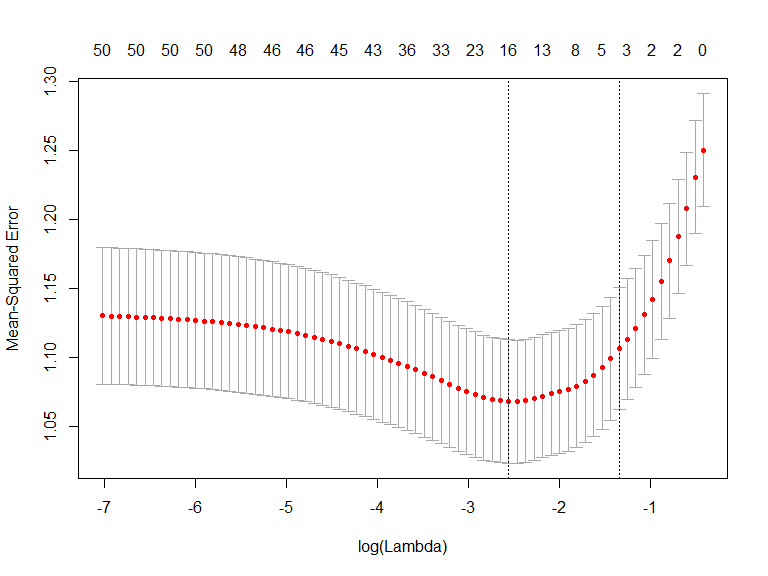## Variables that remain after filtering ##
res0$filter.vars #>  "X1" "X2" "X3" "X5" "X7" "X8" "X10" "X12" "X16" "X18" "X24" #>  "X26" "X31" "X40" "X46" "X50" # All predictive variables (X1,X2) and prognostic variables (X3,X5, X7) remains. Results relating to the PLE model include “ple.mod” (model output), “mu.train” (training predictions), and “mu.test” (test predictions) where, for continuous or binary data, predictions are of E(Y|X,A=a) and E(Y|X,A=1)-E(Y|X,A=0). The PLEs, or individual treatment effects, are informative of the overall treatment heterogeneity and can be visualized through built-in density and waterfall plots. PRISM plots are built using “ggplot2”, making it easy to enhance plot visualizations. For example, prob.PLE = mean(I(res0$mu_train$PLE>0)) # Density Plot # plot(res0, type="PLE:density")+geom_vline(xintercept = 0) + geom_text(x=1.5, y=0.4, label=paste("Prob(PLE>0)=", prob.PLE, sep=""))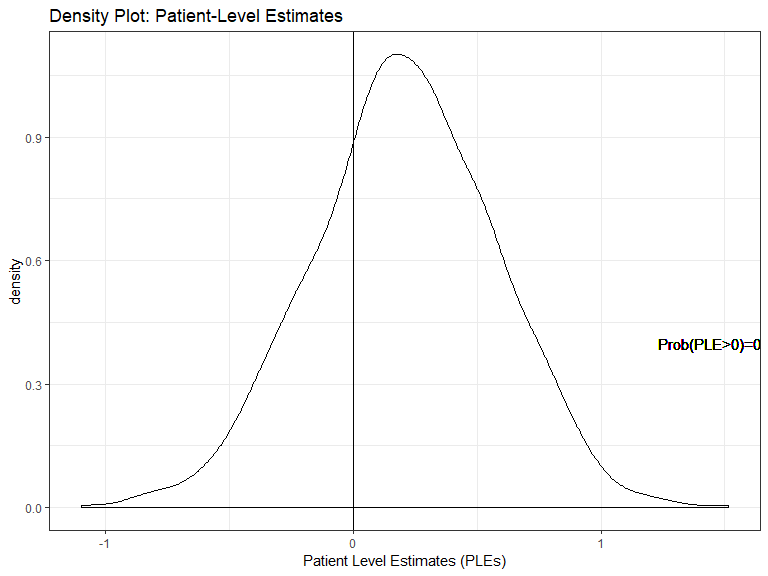# Waterfall Plot # plot(res0, type="PLE:waterfall")+geom_vline(xintercept = 0) + geom_text(x=200, y=1, label=paste("Prob(PLE>0)=", prob.PLE, sep=""))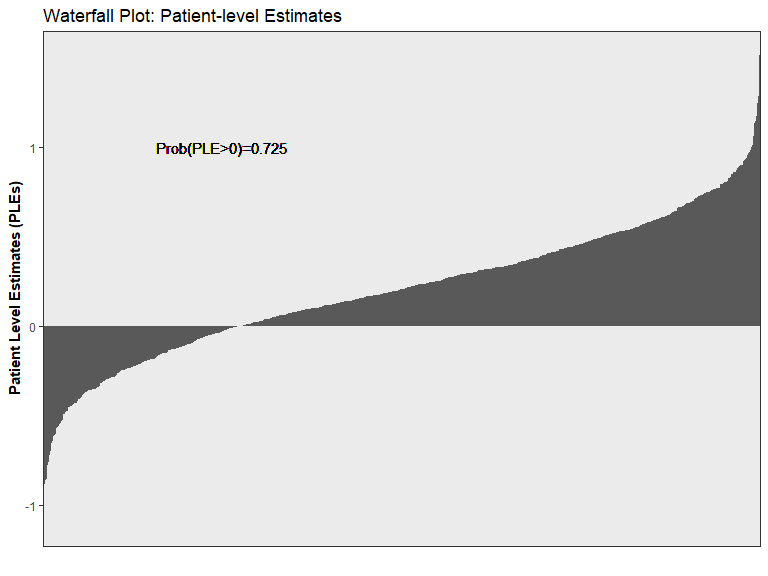Next, the subgroup model (lmtree), identifies 4-subgroups based on varying treatment effects. To visualize this, we use the built-in plotting options for lmtree (within the powerful partykit R package). By plotting the subgroup model object (“submod.fit$mod”)“, we see that partitions are made through X1 (predictive) and X2 (predictive). At each node, parameter estimates for node (subgroup) specific OLS models, $$Y\sim \beta_0+\beta_1*A$$. For example, patients in nodes 4 and 6 have estimated treatment effects of 0.47 and 0.06 respectively. Subgroup predictions for the train/test set can be found in the”out.train" and “out.test” data-sets.

plot(res0$submod.fit$mod, terminal_panel = NULL)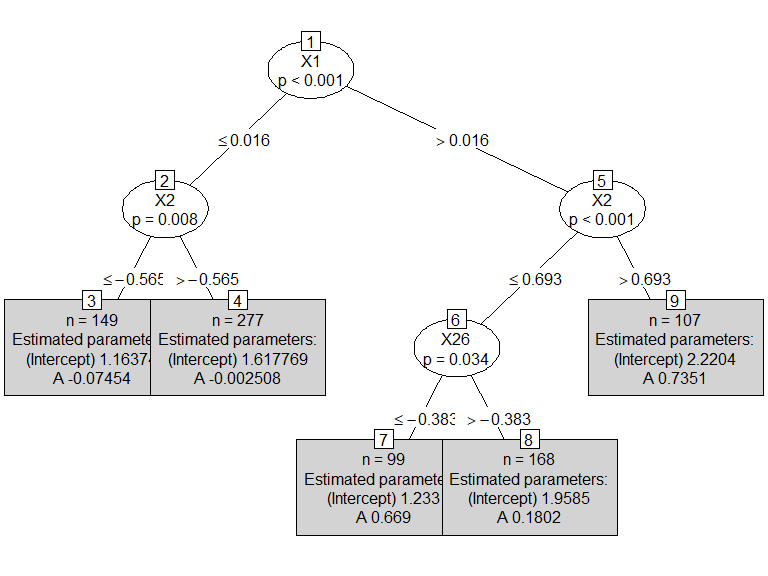table(res0$out.train$Subgrps)
#>
#>   3   4   7   8   9
#> 149 277  99 168 107
table(res0$out.test$Subgrps)
#>
#>   3   4   7   8   9
#> 149 277  99 168 107

These estimates tend to be overly positive or negative, as the same data that trains the subgroup model is used to estimate the treatment effects. Resampling, such as bootstrapping, can generally be used for“de-biased”treatment effect estimates and obtain valid inference. An alternative approach without resampling is the use the PLEs for parameter estimation and inference (param=“param_ple”). Currently, “param_ple” is only implemented for continuous and binary data. For the overall population and each subgroup ($$s=0,,,.S$$), parameter estimates are obtained by averaging the PLEs:

$\hat{\theta}_k = \frac{1}{n_k} \sum_{i \in S_k} {\theta}(x_i)$ For SEs / CIs, we utilize “pseudo-outcomes”: $Y^{\star}_i = \frac{AY - (A-P(A|X))E(Y|A=1,X)}{P(A|X)} - \frac{(1-A)Y - (A-P(A|X))E(Y|A=0,X)}{1-P(A|X)}$ Note that $$E(Y^{\star}_i)=E(Y|A=1,X)-E(Y|A=0,X)$$ and $$E(n_k^{-1}\sum_{i \in S_k} Y^{\star}_i)= E(Y|A=1, X \in S_k)-E(Y|A=0, X \in S_k)$$. Next: $SE(\hat{\theta}_k) = \sqrt{ n_k ^ {-2} \sum_{i \in S_k} \left( Y^{\star}_i-\hat{\theta}(x_i) \right)^2 }$ CIs can then be formed using t- or Z-intervals. For example, a two-sided 95% Z-interval, $$CI_{\alpha}(\hat{\theta}_{k}) = \left[\hat{\theta}_{k} \pm 1.96*SE(\hat{\theta}_k) \right]$$

Moving back to the PRISM outputs, for any of the provided “param” options, a key output is the object “param.dat”. By default, “param.dat” contain point-estimates, standard errors, lower/upper confidence intervals (depends on alpha_s and alpha_ovrl) and p-values. The plot() function can then creates a forest plot based on the “param.dat” object.

## Overall/subgroup specific parameter estimates/inference
res0$param.dat #> Subgrps N estimand est SE LCL #> 1 0 800 E(Y|A=0) 1.63776866 0.04569877 1.54806484 #> 2 0 800 E(Y|A=1) 1.84286992 0.04866618 1.74734124 #> 3 0 800 E(Y|A=1)-E(Y|A=0) 0.20510125 0.06328619 0.08087441 #> 4 3 149 E(Y|A=0) 1.28263595 0.11176578 1.06177307 #> 5 3 149 E(Y|A=1) 1.31794039 0.10980919 1.10094399 #> 6 3 149 E(Y|A=1)-E(Y|A=0) 0.03530445 0.15439447 -0.26979794 #> 7 4 277 E(Y|A=0) 1.60937815 0.06712763 1.47723094 #> 8 4 277 E(Y|A=1) 1.67799950 0.07693142 1.52655259 #> 9 4 277 E(Y|A=1)-E(Y|A=0) 0.06862135 0.10088192 -0.12997442 #> 10 7 99 E(Y|A=0) 1.59590822 0.14128296 1.31553678 #> 11 7 99 E(Y|A=1) 1.92893256 0.13048087 1.66999752 #> 12 7 99 E(Y|A=1)-E(Y|A=0) 0.33302434 0.18994596 -0.04391723 #> 13 8 168 E(Y|A=0) 1.76751942 0.08920057 1.59141332 #> 14 8 168 E(Y|A=1) 2.04252025 0.09776124 1.84951308 #> 15 8 168 E(Y|A=1)-E(Y|A=0) 0.27500084 0.13063657 0.01708886 #> 16 9 107 E(Y|A=0) 2.04080610 0.13504592 1.77306443 #> 17 9 107 E(Y|A=1) 2.60756289 0.11565560 2.37826441 #> 18 9 107 E(Y|A=1)-E(Y|A=0) 0.56675679 0.17669715 0.21643749 #> UCL pval #> 1 1.7274725 1.879424e-168 #> 2 1.9383986 1.723772e-180 #> 3 0.3293281 1.241074e-03 #> 4 1.5034988 3.314673e-22 #> 5 1.5349368 1.324710e-23 #> 6 0.3404068 8.194458e-01 #> 7 1.7415254 1.972697e-69 #> 8 1.8294464 5.334423e-62 #> 9 0.2672171 4.969388e-01 #> 10 1.8762797 1.916696e-19 #> 11 2.1878676 1.077698e-26 #> 12 0.7099659 8.268452e-02 #> 13 1.9436255 1.028800e-45 #> 14 2.2355274 1.856343e-48 #> 15 0.5329128 3.678032e-02 #> 16 2.3085478 3.359840e-28 #> 17 2.8368614 3.054161e-42 #> 18 0.9170761 1.770848e-03 ## Forest plot: Overall/subgroup specific parameter estimates (CIs) plot(res0, type="forest")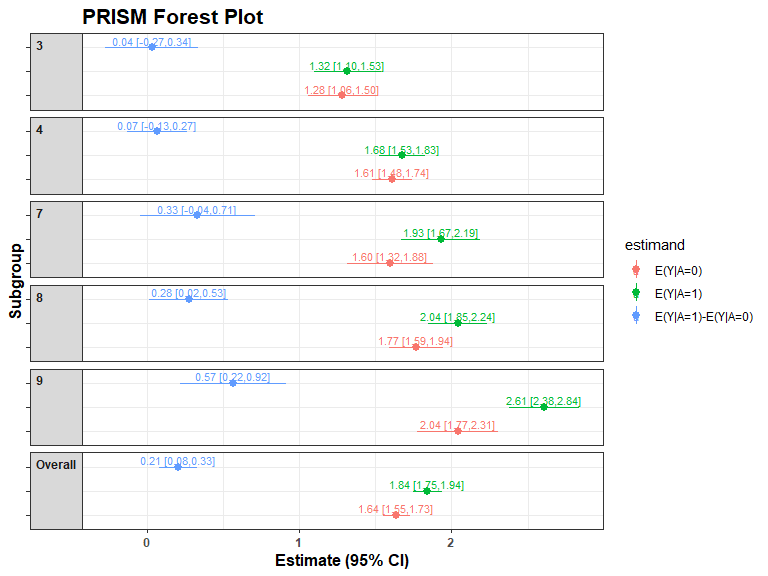The lmtree tree plot can also be modified to include the PLE based parameter estimates: param.dat = res0$param.dat[res0$param.dat$Subgrps>0 &
res0$param.dat$estimand=="E(Y|A=1)-E(Y|A=0)",]
param.dat$est = with(param.dat, sprintf("%.2f",round(est,2))) param.dat$CI = with(param.dat, paste("[", sprintf("%.2f",round(param.dat$LCL,2)),",", sprintf("%.2f",round(param.dat$UCL,2)),"]",sep=""))
smod = res0$submod.fit$mod
smod_node <- as.list(smod$node) for(i in 1:nrow(param.dat)){ smod_node[[param.dat[i,1]]]$info$est <- param.dat$est[i]
smod_node[[param.dat[i,1]]]$info$CI <-  param.dat$CI[i] } smod$node <- as.partynode(smod_node)
plot(smod, terminal_panel = node_terminal, tp_args = list(
FUN = function(node) c( paste("n =", node$nobs), "E(Y|A=1)-E(Y|A=0)", paste(node$est, node$CI) ) ) )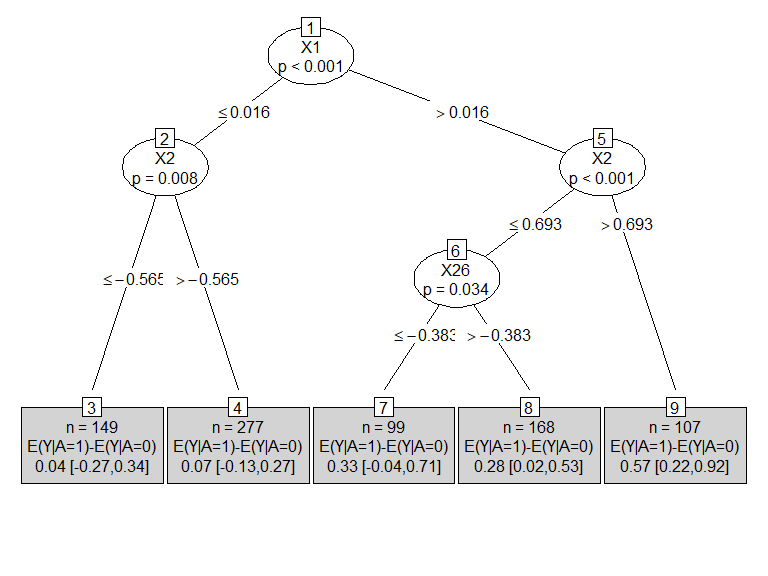PLE Heatmaps can also be generated from PRISM outputs. To do this, based on a grid of values (with up to three variables), PLEs are estimated for each patient by fixing the grid variables to specific values. We then average the PLEs to obtain a point-estimate for each specific set of grid values, and can likewise calculate probabilities. See below; note that the heatmap is also consistent with the truth (treatment benefit for $$X_1>0, X_2>0$$ patients). grid.data = expand.grid(X1 = seq(min(X$X1), max(X$X1), by=0.30), X2 = seq(min(X$X2), max(X$X2), by=0.30)) plot(res0, type="heatmap", grid.data = grid.data) #>$heatmap.est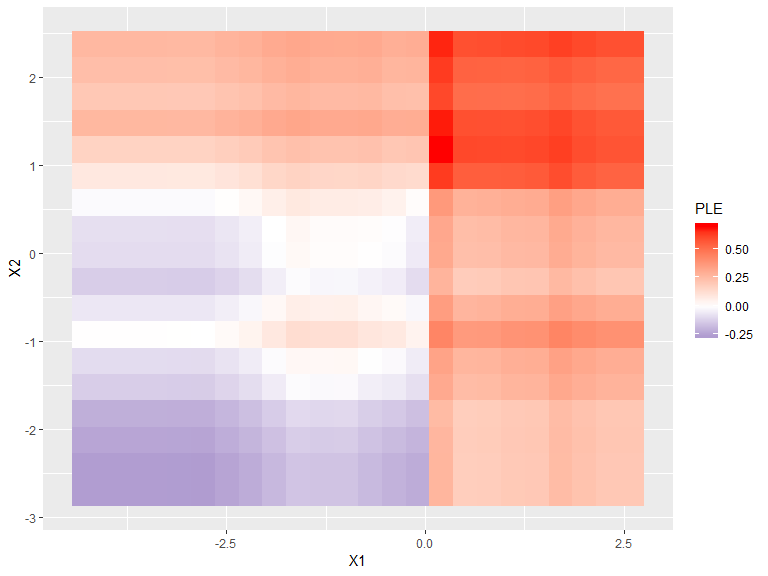#>
#> $heatmap.prob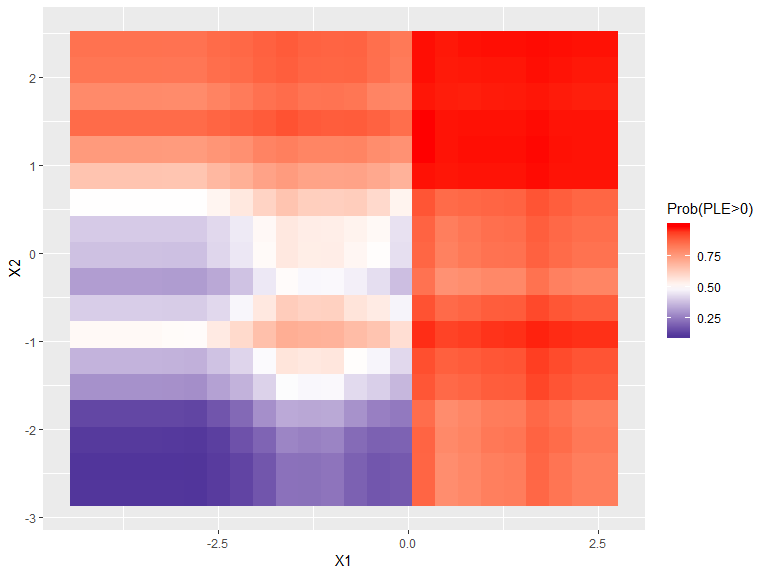The hyper-parameters for the individual steps of PRISM can also be easily modified. For example, “filter_glmnet” by default selects covariates based on “lambda.min”, “ple_ranger” requires nodes to contain at least 10% of the total observations, and “submod_lmtree” requires nodes to contain at least 10% of the total observations. To modify this: # PRISM Default: filter_glmnet, ple_ranger, submod_lmtree, param_ple # # Change hyper-parameters # res_new_hyper = PRISM(Y=Y, A=A, X=X, filter.hyper = list(lambda="lambda.1se"), ple.hyper = list(min.node.pct=0.05), submod.hyper = list(minsize=200), verbose=FALSE) plot(res_new_hyper$submod.fit$mod) # Plot subgroup model results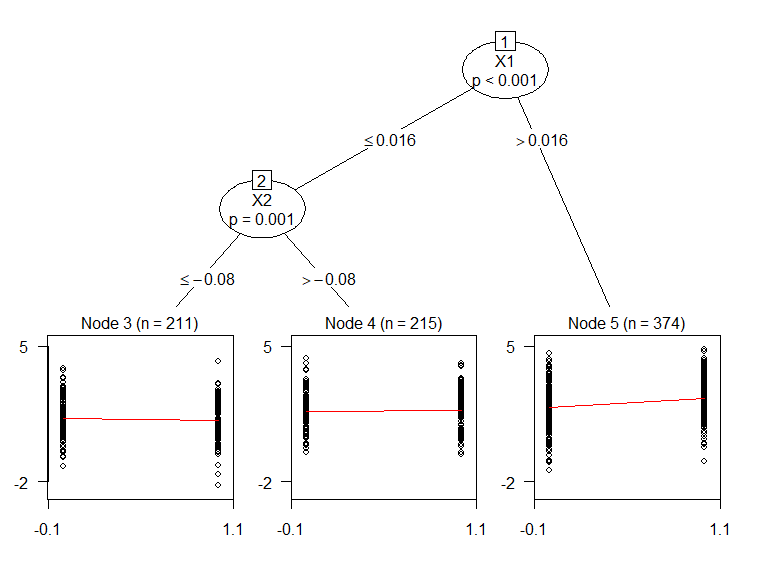plot(res_new_hyper) # Forest plot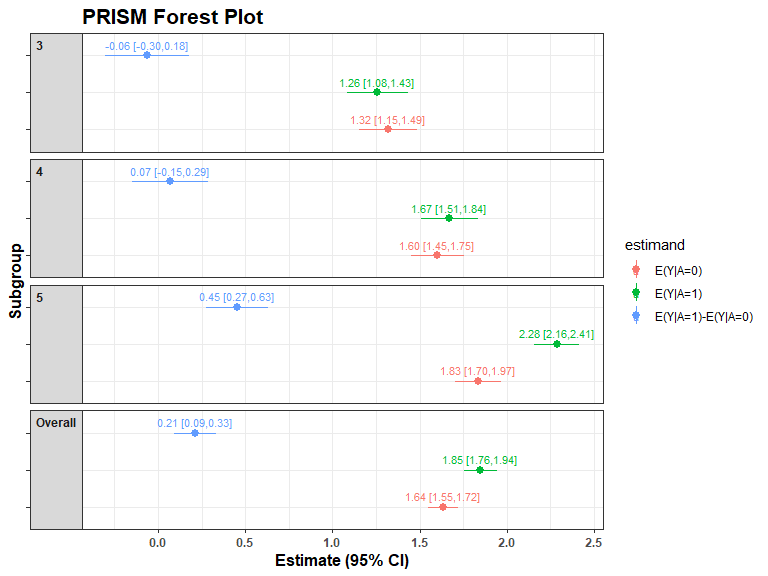Resampling is also a feature in PRISM. Both bootstrap (resample=“Bootstrap”) and permutation (resample=“Permutation”) based-resampling are included; this can be stratified by the discovered subgroups (default: stratify=TRUE). Resampling can be useful for obtaining valid inference and estimating posterior probabilities. In practice, the number of resamples should be larger (i.e R=1000). library(ggplot2) library(dplyr) res_boot = PRISM(Y=Y, A=A, X=X, resample = "Bootstrap", R=50, verbose=FALSE) #> Warning: package 'bindrcpp' was built under R version 3.5.2 # Plot of distributions # plot(res_boot, type="resample", estimand = "E(Y|A=1)-E(Y|A=0)")+geom_vline(xintercept = 0)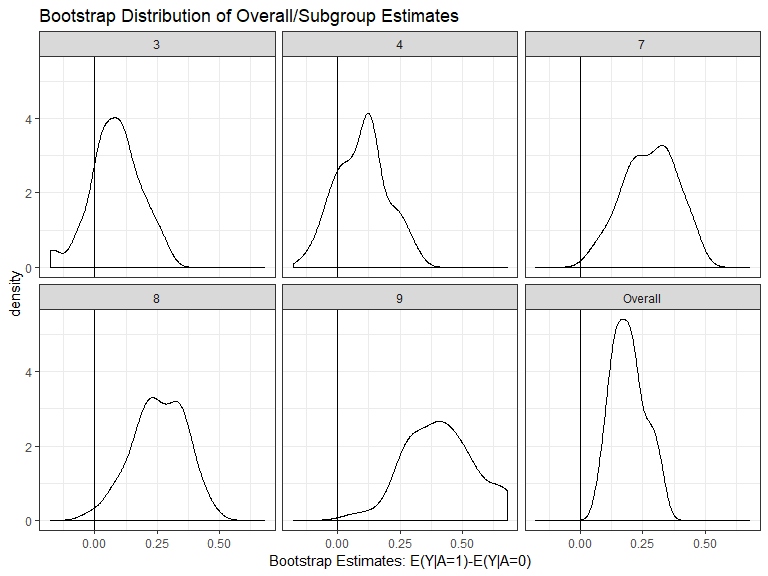# Example: Survival Outcome with Binary Treatment Survival outcomes are also allowed in PRISM. The default settings use glmnet to filter (“filter_glmnet”), ranger patient-level estimates (“ple_ranger”; for survival, the output is the restricted mean survival time treatment difference), “submod_weibull”" (MOB with weibull loss function) for subgroup identification, and param_cox (subgroup-specific cox regression models). Another subgroup option is to use “submod_ctree”“, which uses the conditional inference tree (ctree) algorithm to find subgroups; this looks for partitions irrespective of treatment assignment and thus corresponds to finding prognostic effects. library(survival) library(ggplot2) # Load TH.data (no treatment; generate treatment randomly to simulate null effect) ## data("GBSG2", package = "TH.data") surv.dat = GBSG2 # Design Matrices ### Y = with(surv.dat, Surv(time, cens)) X = surv.dat[,!(colnames(surv.dat) %in% c("time", "cens")) ] A = rbinom( n = dim(X), size=1, prob=0.5 ) # Default: filter_glmnet ==> submod_weibull (MOB with Weibull) ==> param_cox (Cox regression) res_weibull1 = PRISM(Y=Y, A=A, X=X) #> Observed Data #> Filtering: filter_glmnet #> PLE: ple_ranger #> Subgroup Identification: submod_weibull #> Parameter Estimation: param_cox plot(res_weibull1, type="PLE:waterfall")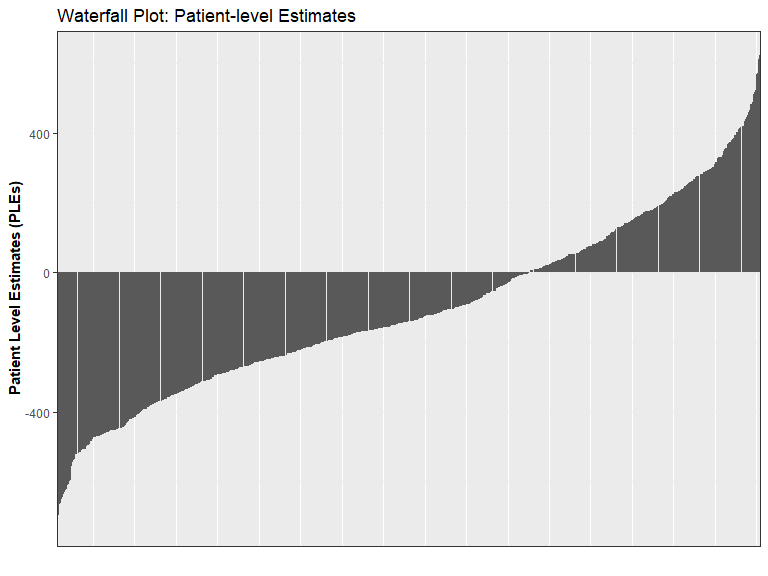plot(res_weibull1$submod.fit$mod)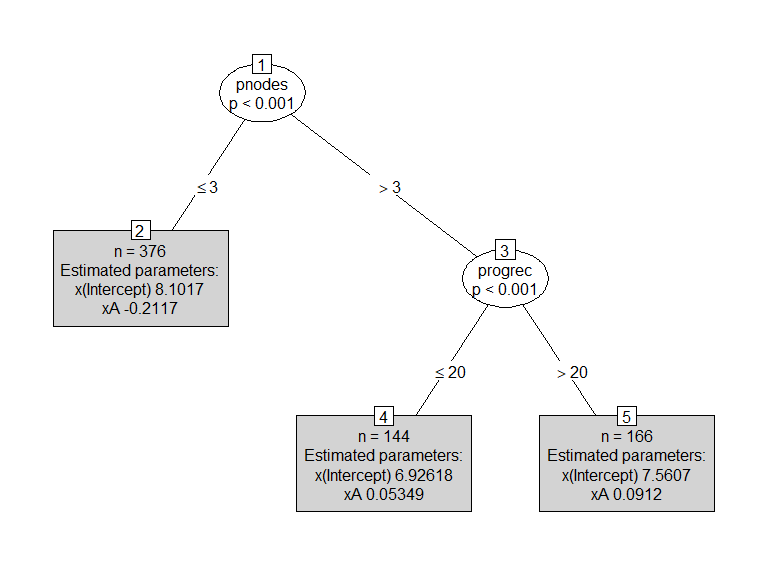plot(res_weibull1)+ylab("HR [95% CI]")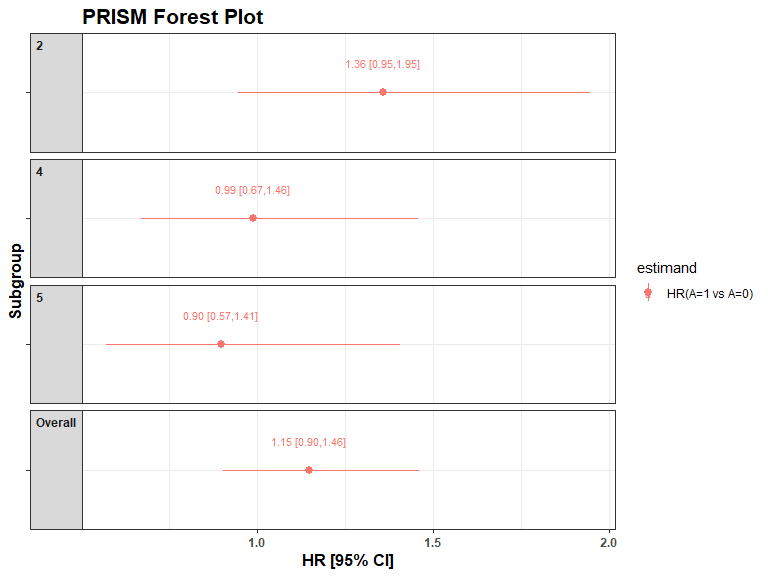# PRISM: filter_glmnet ==> submod_ctree ==> param_cox (Cox regression) # res_ctree1 = PRISM(Y=Y, A=A, X=X, ple=NULL, submod = "submod_ctree") #> Observed Data #> Filtering: filter_glmnet #> PLE: ple_ranger #> Subgroup Identification: submod_ctree #> Parameter Estimation: param_cox plot(res_ctree1$submod.fit$mod)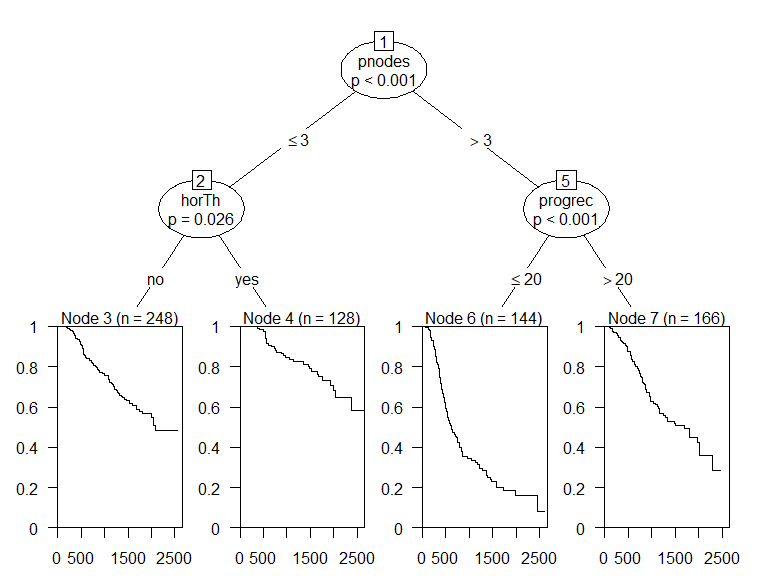plot(res_ctree1)+ylab("HR [95% CI]")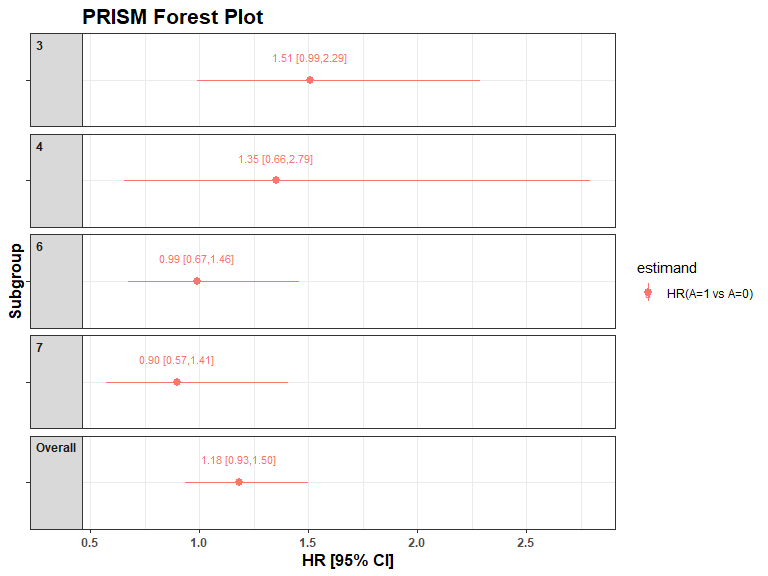# User-Created Models One advantage of PRISM is the flexibility to adjust each step of the algorithm and also to input user-created functions/models. This facilitates faster testing and experimentation. First, let’s simulate the continuous data again. library(StratifiedMedicine) dat_ctns = generate_subgrp_data(family="gaussian") Y = dat_ctns$Y
X = dat_ctns$X # 50 covariates, 46 are noise variables, X1 and X2 are truly predictive A = dat_ctns$A # binary treatment, 1:1 randomized 

Next, let’s going over the basic function template for the “filter”, “ple”, “submod”, and “param” steps in PRISM. For “filter,” consider the lasso:

filter_lasso = function(Y, A, X, lambda="lambda.min", family="gaussian", ...){
require(glmnet)
## Model matrix X matrix #
X = model.matrix(~. -1, data = X )

##### Elastic Net on estimated ITEs #####
set.seed(6134)
if (family=="survival") { family = "cox"  }
mod <- cv.glmnet(x = X, y = Y, nlambda = 100, alpha=1, family=family)

### Extract filtered variable based on lambda ###
VI <- coef(mod, s = lambda)[,1]
VI = VI[-1]
filter.vars = names(VI[VI!=0])
return( list(filter.vars=filter.vars) )
}

Note that the filter uses the observed data (Y,A,X), which are required inputs, and outputs an object called “filter.vars.” This needs to contain the variable names of the variables that pass the filtering step. An option to change lambda, which can change which variables remain after filtering (lambda.min keeps more, lambda.1se keeps less), while not required, is included. This can then be adjusted in the “filter.hyper” argument in PRISM.

For “ple,” consider treatment-specific random forest (ranger) models.

ple_ranger_mtry = function(Y, A, X, Xtest, mtry=5, ...){
require(ranger)
## Split data by treatment ###
train0 =  data.frame(Y=Y[A==0], X[A==0,])
train1 =  data.frame(Y=Y[A==1], X[A==1,])
# Trt 0 #
mod0 <- ranger(Y ~ ., data = train0, seed=1, mtry = mtry)
# Trt 1 #
mod1 <- ranger(Y ~ ., data = train1, seed=2, mtry = mtry)
mods = list(mod0=mod0, mod1=mod1)
## Predictions: Train/Test ##
mu_train = data.frame( mu1 =  predict(mod1, data = X)$predictions, mu0 = predict(mod0, data = X)$predictions)
mu_train$PLE = with(mu_train, mu1 - mu0 ) mu_test = data.frame( mu1 = predict(mod1, data = Xtest)$predictions,
mu0 = predict(mod0, data = Xtest)$predictions) mu_test$PLE = with(mu_test, mu1 - mu0 )
res = list(mods=mods, mu_train=mu_train, mu_test=mu_test)
class(res) = "ple_ranger_mtry"
return( list(mods=mods, mu_train=mu_train, mu_test=mu_test))
}

For the “ple” model, the only required arguments are the observed data (Y,A,X) and Xtest. By default, if Xtest is not provided in PRISM, it uses the training X instead. The only required outputs are mu_train and mu_test, which include a vector/column named “PLE” (Patient-level estimates). In this example, we allow “mtry” (number of variables randomly selected at each split) to vary and can be altered in the “ple.hyper” argument in PRISM.

For “submod”, consider a modified version of “submod_lmtree” where we search for predictive effects only. By default, “submod_lmtree” searches for prognostic and/or predictive effects.

submod_lmtree_pred = function(Y, A, X, Xtest, mu_train, ...){
require(partykit)
## Fit Model ##
mod <- lmtree(Y~A | ., data = X, parm=2) ##parm=2 focuses on treatment interaction #
##  Predict Subgroups for Train/Test ##
Subgrps.train = as.numeric( predict(mod, type="node") )
Subgrps.test = as.numeric( predict(mod, type="node", newdata = Xtest) )
## Predict E(Y|X=x, A=1)-E(Y|X=x,A=0) ##
pred.train = predict( mod, newdata = data.frame(A=1, X) ) -
predict( mod, newdata = data.frame(A=0, X) )
pred.test =  predict( mod, newdata = data.frame(A=1, Xtest) ) -
predict( mod, newdata = data.frame(A=0, Xtest) )
## Return Results ##
return(  list(mod=mod, Subgrps.train=Subgrps.train, Subgrps.test=Subgrps.test,
pred.train=pred.train, pred.test=pred.test) )
}

For the “submod” model, the only required arguments are the observed data (Y,A,X) and Xtest. By default, if Xtest is not provided in PRISM, it uses the training X instead. Note that “mu_train” is included as an argument here. If a subgroup model uses the PLEs to form groups, then this is a required argument. Required outputs include mod (the subgroup model) and Subgrps.train/Subgrps.test (predicted subgroups in train/test set). This function does also include predicted treatment effects for train/test; these aren’t required but are needed if the subgroup model is explicitly used in the final parameter estimation step.

Lastly, the “param” model outputs parameter estimates and variability metrics for the overall population and discovered subgroups. Just for demonstration, one option could be to fit subgroup-specific robust regression (M-estimator) models.


### Robust linear Regression: E(Y|A=1) - E(Y|A=0) ###
param_rlm = function(Y, A, X, mu_hat, Subgrps, alpha_ovrl, alpha_s, ...){
require(MASS)
indata = data.frame(Y=Y,A=A, X)
mod.ovrl = rlm(Y ~ A , data=indata)
param.dat0 = data.frame( Subgrps=0, N = dim(indata),
estimand = "E(Y|A=1)-E(Y|A=0)",
est = summary(mod.ovrl)$coefficients[2,1], SE = summary(mod.ovrl)$coefficients[2,2] )
param.dat0$LCL = with(param.dat0, est-qt(1-alpha_ovrl/2, N-1)*SE) param.dat0$UCL = with(param.dat0, est+qt(1-alpha_ovrl/2, N-1)*SE)
param.dat0$pval = with(param.dat0, 2*pt(-abs(est/SE), df=N-1) ) ## Subgroup Specific Estimate ## looper = function(s){ rlm.mod = tryCatch( rlm(Y ~ A , data=indata[Subgrps==s,]), error = function(e) "param error" ) n.s = dim(indata[Subgrps==s,]) est = summary(rlm.mod)$coefficients[2,1]
SE = summary(rlm.mod)$coefficients[2,2] LCL = est-qt(1-alpha_ovrl/2, n.s-1)*SE UCL = est+qt(1-alpha_ovrl/2, n.s-1)*SE pval = 2*pt(-abs(est/SE), df=n.s-1) return( c(est, SE, LCL, UCL, pval) ) } S_levels = as.numeric( names(table(Subgrps)) ) S_N = as.numeric( table(Subgrps) ) param.dat = lapply(S_levels, looper) param.dat = do.call(rbind, param.dat) param.dat = data.frame( S = S_levels, N=S_N, estimand="E(Y|A=1)-E(Y|A=0)", param.dat) colnames(param.dat) = c("Subgrps", "N", "estimand", "est", "SE", "LCL", "UCL", "pval") param.dat = rbind( param.dat0, param.dat) return( param.dat ) } Finally, let’s put it all together directly into PRISM:  res_user1 = PRISM(Y=Y, A=A, X=X, family="gaussian", filter="filter_lasso", ple = "ple_ranger_mtry", submod = "submod_lmtree_pred", param="param_rlm") #> Observed Data #> Filtering: filter_lasso #> Loading required package: glmnet #> Warning: package 'glmnet' was built under R version 3.5.3 #> Loading required package: Matrix #> Loading required package: foreach #> Warning: package 'foreach' was built under R version 3.5.2 #> Loaded glmnet 2.0-18 #> PLE: ple_ranger_mtry #> Loading required package: ranger #> Warning: package 'ranger' was built under R version 3.5.2 #> Subgroup Identification: submod_lmtree_pred #> Parameter Estimation: param_rlm #> Loading required package: MASS #> #> Attaching package: 'MASS' #> The following object is masked from 'package:dplyr': #> #> select ## variables that remain after filtering ## res_user1$filter.vars
#>   "X1"  "X2"  "X3"  "X5"  "X7"  "X8"  "X10" "X12" "X16" "X18" "X24"
#>  "X26" "X31" "X40" "X46" "X50"
## Subgroup model: lmtree searching for predictive only ##
plot(res_user1$submod.fit$mod)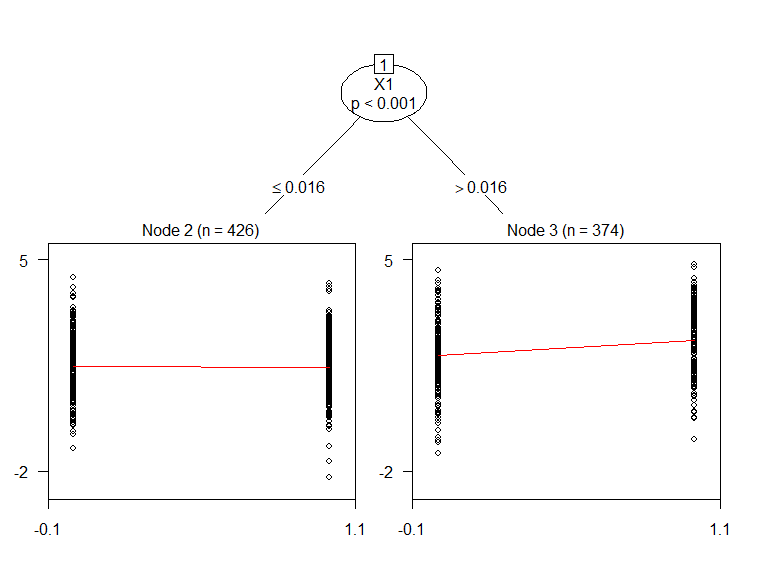## Parameter estimates/inference
res_user1\$param.dat
#>   Subgrps   N          estimand          est         SE         LCL
#> 1       0 800 E(Y|A=1)-E(Y|A=0)  0.229913662 0.08114137  0.07063823
#> 2       2 426 E(Y|A=1)-E(Y|A=0) -0.004165125 0.10352507 -0.20765001
#> 3       3 374 E(Y|A=1)-E(Y|A=0)  0.506836561 0.11525208  0.28021130
#>         UCL         pval
#> 1 0.3891891 4.720217e-03
#> 2 0.1993198 9.679263e-01
#> 3 0.7334618 1.429483e-05
## Forest Plot (95% CI) ##
plot(res_user1)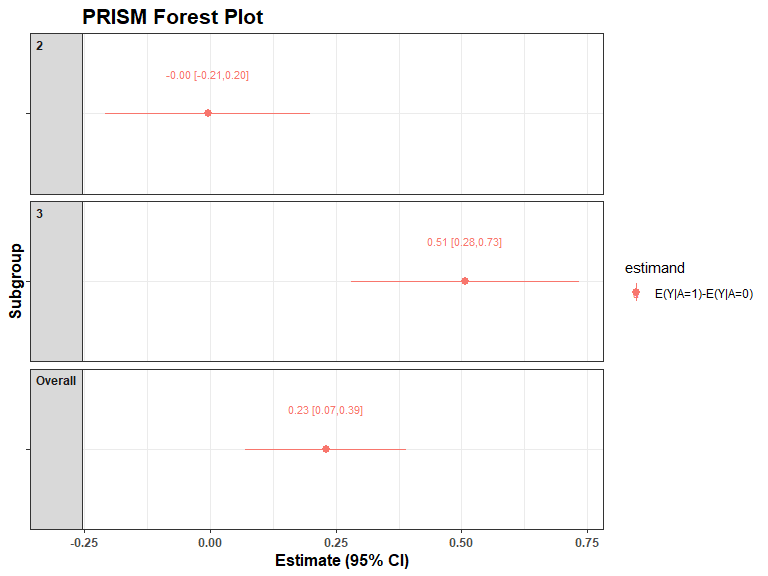# Conclusion

Overall, PRISM is a flexible algorithm that can aid in subgroup detection and exploration of heterogeneous treatment effects. Each step of PRISM is customizable, allowing for fast experimentation and improvement of individual steps. The StratifiedMedicine R package and PRISM will be continually updated and improved. User-feedback will further faciliate improvements.